# NCERT SOLUTIONS FOR CLASS 9 MATHS CHAPTER 5 INTRODUCTION TO EUCLID GEOMETRY

The National Council of Educational Research and Training (NCERT) is an autonomous body of the Indian government that formulates the curricula for schools in India that are governed by the Central Board of Secondary Education (CBSE) and certain state boards. Therefore, students who will be taking the Class 10 tests administered by various boards should consult this NCERT Syllabus in order to prepare for those examinations, which in turn will assist those students get a passing score.

When working through the exercises in the NCERT textbook, if you run into any type of difficulty or uncertainty, you may use the swc NCERT Solutions for class 9 as a point of reference. While you are reading the theory form textbook, it is imperative that you always have notes prepared. You should make an effort to understand things from the very beginning so that you may create a solid foundation in the topic. Use the NCERT as your parent book to ensure that you have a strong foundation. After you have finished reading the theoretical section of the textbook, you should go to additional reference books.

### NCERT Solutions for Class 9 Maths Exercise 5.1

Question 1. Which of the following statements are true and which are false? Give reasons for your answers.
(i) Only one line can pass through a single point.
(ii) There are an infinite number of lines which pass through two distinct points.
(iii) A terminated line can be produced indefinitely on both the sides.
(iv) If two circles are equal, then their radii are equal.
(v) In figure, if AB – PQ and PQ = XY, then AB = XY.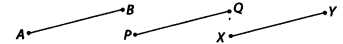Solution :
(i) False
Reason : If we mark a point O on the surface of a paper. Using pencil and scale, we can draw infinite number of straight lines passing
through O.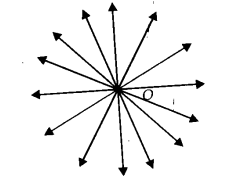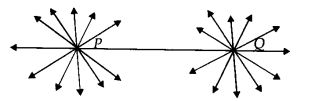(ii) False
Reason : In the following figure, there are many straight lines passing through P. There are many lines, passing through Q. But there is one and only one line which is passing through P as well as Q.

(iii) True
Reason: The postulate 2 says that “A terminated line can be produced indefinitely.”

(iv) True
Reason : Superimposing the region of one circle on the other, we find them coinciding. So, their centres and boundaries coincide.
Thus, their radii will coincide or equal.

(v) True
Reason : According to Euclid’s axiom, things which are equal to the same thing are equal to one another.

Question 2. Give a definition for each of the following terms. Are there other terms that need to be defined first? What are they and how might you define them?
(i) Parallel lines
(ii) Perpendicular lines
(iii) Line segment
(v) Square

Solution: Yes, we need to have an idea about the terms like point, line, ray, angle, plane, circle and quadrilateral, etc. before defining the required terms.
Definitions of the required terms are given below :

(i) Parallel Lines :
Two lines l and m in a plane are said to be parallel, if they have no common point and we write them as l ॥ m.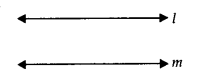(ii) Perpendicular Lines :
Two lines p and q lying in the same plane are said to be perpendicular if they form a right angle and we write them as p ⊥ q.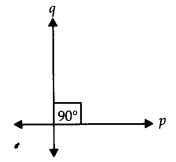(iii) Line Segment :
A line segment is a part of line and having a definite length. It has two end-points. In the figure, a line segment is shown having end points A and B. It is written as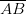or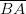.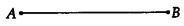(iv) Radius of a circle :
The distance from the centre to a point on the circle is called the radius of the circle. In the figure, P is centre and Q is a point on the circle, then PQ is the radius.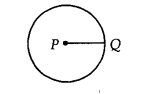(v) Square :
A quadrilateral in which all the four angles are right angles and all the four sides are equal is called a square. Given figure, PQRS is a square.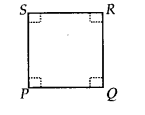Question 3. Consider two ‘postulates’ given below
(i) Given any two distinct points A and B, there exists a third point C which is in between A and B.
(ii) There exist atleast three points that are not on the same line.
Do these postulates contain any undefined terms? Are these postulates consistent? Do they follow from Euclid’s postulates? Explain.

Solution: Yes, these postulates contain undefined terms such as ‘Point and Line’. Also, these postulates are consistent because they deal with two different situations as
(i) says that given two points A and B, there is a point C lying on the line in between them. Whereas
(ii) says that, given points A and B, you can take point C not lying on the line through A and B.
No, these postulates do not follow from Euclid’s postulates, however they follow from the axiom, “Given two distinct points, there is a unique line that passes through them.”

Question 4. If a point C lies between two points A and B such that AC = BC, then prove that AC = AB, explain by drawing the figure.

Solution:
We have,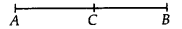AC = BC [Given]
∴ AC + AC = BC + AC
[If equals added to equals then wholes are equal]
or 2AC = AB [∵ AC + BC = AB]
or AC =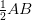Question 5. In question 4, point C is called a mid-point of line segment AB. Prove that every line segment has one and only one mid-point.

Solution:
Let the given line AB is having two mid points ‘C’ and ‘D’.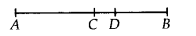AC =……(i)
and AD =……(ii)
Subtracting (i) from (ii), we have
AD – AC =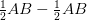or AD – AC = 0 or CD = 0
∴ C and D coincide.
Thus, every line segment has one and only one mid-point.

Question 6. In figure, if AC = BD, then prove that AB = CD.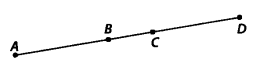Solution:
Given: AC = BD
⇒ AB + BC = BC + CD
Subtracting BC from both sides, we get
AB + BC – BC = BC + CD – BC
[When equals are subtracted from equals, remainders are equal]
⇒ AB = CD

Question 7. Why is axiom 5, in the list of Euclid’s axioms, considered a ‘universal truth’? (Note that, the question is not about the fifth postulate.)

Solution: As statement is true in all the situations. Hence, it is considered a ‘universal truth.’

### NCERT Solutions for Class 9 Maths Exercise 5.2

Question 1. How would you rewrite Euclid’s fifth postulate so that it would be easier to understand?

Solution:
We need to rewrite Euclid’s fifth postulate so that it is easier to understand.

We know that Euclid’s fifth postulate states that “No intersection of lines will take place when the sum of the measures of the interior angles on the same side of the falling line is exactly 180°.”

We know that Play fair’s axiom states that “For every line l and for every point P not lying on l, there exists a unique line m passing through P and parallel to l”.

The above mentioned Play fair’s axiom is easier to understand in comparison to the Euclid’s fifth postulate.

Let us consider a line l that passes through a point p and another line m. Let these lines be at a same plane.

Let us consider the perpendicular CD on l and FE on m.

From the above figure, we can conclude that CD = EF.

Therefore, we can conclude that the perpendicular distance between lines m and l will be constant throughout, and the lines m and l will never meet each other or in other words, we can say that the lines m and l are equidistant from each other.

Question 2. Does Euclid’s fifth postulate imply the existence of parallel lines? Explain.

Solution:
We need to verify whether Euclid’s fifth postulate imply the existence of parallel lines or not.

The answer to the above statement is Yes.

Let us consider two lines m and l.

In the figure given below, we can conclude that the lines m and l will intersect further.

From the figure, we can conclude that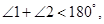and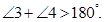We know that Euclid’s fifth postulate states that “No intersection of lines will take place when the sum of the measures of the interior angles on the same side of the falling line is exactly 180°.”

Let us consider lines l and m.

From the above figure, we can conclude that lines l and m will never intersect from either side.

Therefore, we can conclude that the lines l and m are parallel.

## Conclusions for NCERT SOLUTIONS FOR CLASS 9 MATHS CHAPTER 5 INTRODUCTION TO EUCLID GEOMETRY

SWC academic staff has developed NCERT answers for this chapter of the ninth grade mathematics curriculum. We have solutions prepared for all of the exercises of this chapter. The answers, broken down into steps, to all of the questions included in the NCERT textbook’s chapter are provided here. Read this chapter on theory. Be certain that you have read the theory section of this chapter of the NCERT textbook and that you have learnt the formulas for the chapter that you are studying.